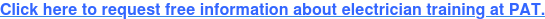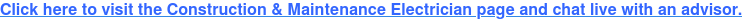# How Much Math do You Need to Become an Electrician?So, how much math do I really need to know to become an electrician?” We get this question all the time, from students who are considering electrician training, but feeling insecure about the math involved.

And it’s no surprise, given the huge number of students who struggle with math in Ontario (and across Canada).

The Globe and Mail recently ran a story on how elementary students’ math scores in Ontario reached a record low in 2017/2018.

Less than half of the province’s Grade 6 students met the current standard for math performance—the worst result in years.

For most of these kids, problems with math will only get worse in high school, as the level of difficulty increases.

By the time graduation rolls around, many are totally “done” with math. They never want to see another algebraic equation or geometry question, as long as they live!

Sound familiar? Have you heard that electricians use a lot of math on the job, and figure you shouldn’t even bother considering this trade?

Before you make up your mind, first understand how electricians actually use math. Take a look at some common applications of math in day-to-day electrical work.

## Using simple math for routine measurements & calculations

Electricians use simple math, like addition, subtraction, multiplication, and division, to perform routine measurements and calculations at work.

You’d be working with fractions, percentages, and decimals to figure out things like room dimensions, wiring lengths, convert watts to kilowatts, and calculate loads.

For example, load calculations typically involve converting percentages to decimals or whole numbers (125% = 1.25).

And then, the electrician would multiply that number by the value of the load to figure out what size circuit breaker or fuse is needed. Sound complicated? It’s really not.

You’ll do hundreds of these simple calculations during your foundations electrician training, and while completing your apprenticeship.

Once you’re applying the math to real-world electrical work, it becomes much easier to grasp than typical questions you’d see in a high school math classroom. Before you know it, it’s second nature.

## Ohm’s Law

If you decide to become an electrician, you will definitely need to master Ohm’s Law—an equation used to study electrical circuits, and figure out voltage, current, and resistance.

The basic equation is E = I X R…which means, Voltage = Current x Resistance.  Here’s an example of a problem you’d see in electrician training, where you’d need to use Ohm’s law to find the answer:

120V supplies a lamp that has a resistance of 192 ohms. What’s the current flow in the circuit?

In this example, you know voltage and you know resistance, but you don’t know current. So, you’d apply Ohm’s Law like this: I = E/R  (Current = 120V/192 ohms). Your answer is I = 0.625A.

Electricians use Ohm’s Law to determine the current of a circuit, voltage drop, power loss, and conductor resistance.

Again, this may seem complex, but it becomes quite routine once you’ve done tons of practice questions.

Ohm’s Law is a good example of the kind of logical thinking, problem solving, and practical math skills you’ll be using as an electrician.

## Trigonometry for Electricians

Trigonometry is a branch of mathematics that deals with the sides and angles of triangles. How is this relevant to electricians, you ask?

One example would be figuring out the correct angle to bend a section of conduit. Electricians use conduit to protect and route electrical wire when wiring a building or structure.

Conduits come in a variety of materials, including metal, plastic, and fiber. Often, conduits must be bent around corners or obstacles—like a step, for example.

Electricians use trigonometry to determine the precise angle they’d need to bend the conduit around the object, and connect it to the circuit.

An example of an electrical-related trig problem would be needing to bend a conduit  around a step that is 4 inches high and 15 inches wide.

In this case, you’d use what’s called the “tangent function” to calculate the exact angle of the bend, which you would find, is 15 degrees.

In electrician training, students learn how to figure out these kinds of equations, and get comfortable applying principles of geometry to solve routine electrical problems.

Remember the Pythagorean Theorem? That’s another basic geometry concept you’ll learn in electrician school, as you work with triangles to understand basic AC power concepts.

From simple addition and subtraction, to algebra and geometry—math is an important part of the electrician skillset.

But remember, you don’t need to be a natural math whiz to become an electrician. Fourth-year apprentice, Jon Kerr, told us in a recent interview, that math was his absolute worst subject in high school:

I was never good at math in high school. I was constantly failing tests, and barely passed grade 12 math. I had dyslexia, but it was never diagnosed, and I really struggled to make it through.

But, once I learned how to ask for help, and starting using better techniques for solving math problems (using pictures, drawing out problems, taking extra time for tests), I did much better.

If I can make it through the math, anyone can! For me, the difference was knowing that the math I learned in electrician training had a real, practical purpose.

At the end of the day, I put in the extra effort and I got it done. My electrician instructors were always helpful and supportive. If you have the drive, and are willing to work hard, you can master these skills.

## Your First Steps to Become an Electrician

Think you’re ready to tackle the math (and many other skills) involved in electrician training, and wondering what steps to take next?